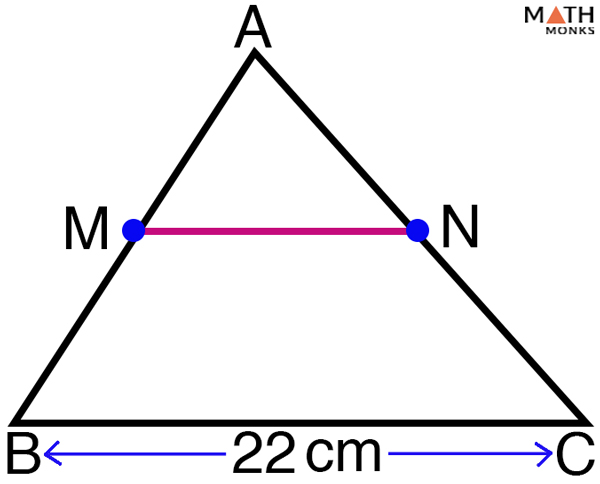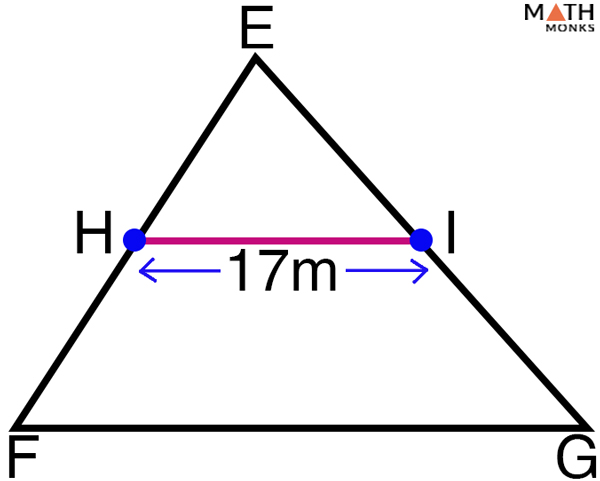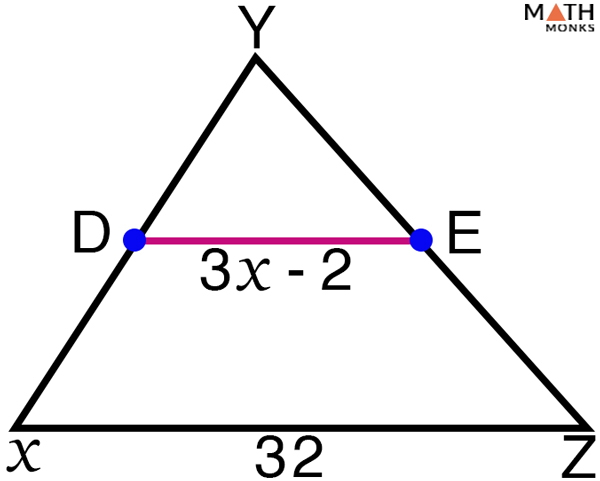# Midsegment of a Triangle

## What is Midsegment of a Triangle

The midsegment of a triangle is a line connecting the midpoints or center of any two (adjacent or opposite) sides of a triangle. It is parallel to the third side and is half the length of the third side.

How Many Midsegments Does a Triangle Have

Since a triangle has three sides, each triangle has 3 midsegments. In the given ∆ABC, DE, EF, and DF are the 3 midsegments. The 3 midsegments form a smaller triangle that is similar to the main triangle. Thus, ∆ABC ~ ∆FED

## Properties

1. It joins the midpoints of 2 sides of a triangle; in ∆ABC, D is the midpoint of AB, E is the midpoint of AC, & F is the midpoint of BC
2. A triangle has 3 possible midsegments; DE, EF, and DF are the three midsegments
3. The midsegment is always parallel to the third side of the triangle; so, DE ∥ BC, EF ∥ AB, and DF ∥ AC
4. The midsegment is always 1/2 the length of the third side; so, DE =1/2 BC, EF =1/2 AB, and DF =1/2  AC

## Formula

### How to Find the Midsegment of a Triangle

The formula to find the length of midsegment of a triangle is given below:

## Triangle Midsegment Theorem

### Proof of Triangle Midsegment Theorem

To prove: DE BC; DE = ½ BC

Proof: A line is drawn parallel to AB, such that when the midsegment DE is produced it meets the parallel line at F

Given: D is the midpoint of AB

E is the midpoint of AC

F is the midpoint of BC

## Converse of Triangle Midsegment Theorem

### Converse of Triangle Midsegment Theorem Proof

To prove: DE is the midsegment of ∆ABC

AD = DB; AE = EC

Proof:

Given: D is the midpoint of AB

E is the midpoint of AC

DE ∥ BC

DE = 1/2 BC

## Solved ExamplesFind MN in the given triangle. Given BC = 22cm, and M, N are the midpoints of AB and AC.

Solution:

As we know, by midpoint theorem,
MN = ½ BC, here BC = 22cm
= ½ x 22 = 11cmGiven G and H are the midpoints and GH = 17m. Find FG.

Solution:

As we know, by the midpoint theorem,
HI = ½ FG, here HI = 17 m
FG = 2 HI = 2 x 17 = 34 mSolve for x in the given triangle. Given that D and E are midpoints.

Solution:

As we know, by midpoint theorem,
DE = ½ XZ, here XZ = 32 units
3x -2 = ½ x 32
3x = 16 + 2 x = 6